﻿ L_GetFunctionalLookupTable | Function References | Raster Imaging C API Help
In This Topic ▼

# L_GetFunctionalLookupTable

#include "l_bitmap.h"

L_LTIMGEFX_API L_INT L_GetFunctionalLookupTable (pLookupTable, uLookupLen, nStart, nEnd, nFactor, uFlags)

Updates a range of entries in the lookup table, based on the specified mathematical function.

## Parameters

### L_INT *pLookupTable

Pointer to the lookup table to be filled by this function. The length of the lookup table is specified in the `uLookupLen` parameter.

### L_UINT uLookupLen

Length of the lookup table pointed to by the `pLookupTable` parameter. It can be any value larger than 0.

### L_INT nStart

Index of the first entry in the lookup table to update. The indices of the table correspond to the intensity values. If the value of this parameter is negative, the corresponding index of pLookupTable is equal to the value of this parameter + uLookupLen. The range of its possible value is between (-uLookupLen/2) and (uLookupLen/2 - 1).

### L_INT nEnd

Index of the last entry in the lookup table to update. The indices of the table correspond to the intensity values. If the value of this parameter is negative, the corresponding index of `pLookupTable` is equal to the value of this parameter + uLookupLen. The range of its possible value is between (-uLookupLen/2) and (`uLookupLen`/2 - 1).

### L_INT nFactor

Value that indicates the factor to be applied in the function operation specified in the `uFlags` parameter. This parameter is used only if uFlags is FLT_EXP, FLT_SIGMOID or FLT_LN. If FLT_EXP or FLT_SIGMOID is set in uFlags the value of this parameter can be any integer (+/-). If FLT_LN flag is set, its value should be >= 0. However, if nFactor = 0 the lookup table will be filled linearly from `nStart` to `nEnd`, regardless of the value set in uFlags.

### L_UINT uFlags

Flag that indicates the function used to update the lookup table and whether the `pLookupTable` should contain signed or unsigned data.

#### Flags indicate the function used to update the lookup table:

Value Meaning
FLT_EXP [0x0000] Apply the exponential function.
FLT_LN [0x0001] Apply the natural logarithm function.
FLT_LINEAR [0x0002] Apply the linear function.
FLT_SIGMOID [0x0003] Apply the sigmoid function.

#### Flag indicates whether the pLookupTable should contain signed or unsigned data:

Value Meaning
FLT_SIGNED [0x0010] pLookupTable should contain signed data.

#### Returns

Value Meaning
SUCCESS The function was successful.
< 1 An error occurred. Refer to Return Codes.

## Comments

This function will update the lookup table array using the predefined function specified in the uFlags parameter. In most cases, this function is used with the L_RemapBitmapIntensity function.

If the value of (nEnd - nStart) exceeds the `uLookupLen`, the function returns ERROR_INV_PARAMETER error code.

The `nFactor` parameter is used for log, exp and sigmoid functions only. If nFactor = 0 the function performs a linear interpolation between the two points `nStart` and `nEnd` and stores the results in the lookup table, regardless of the value set in uFlags.

If `uFlags` is FLT_EXP the value of nFactor modifies the lookup table values (see figure below) according to the following equations: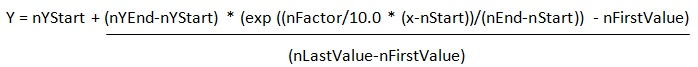Where:

• nYStart = The Lookup table value at `nStart`
• nYEnd = The Lookup table value at `nEnd`
• nFirstValue = exp(0)
• nLastValue = exp(nFactor/10.0)
• x = the intensity value of the selected point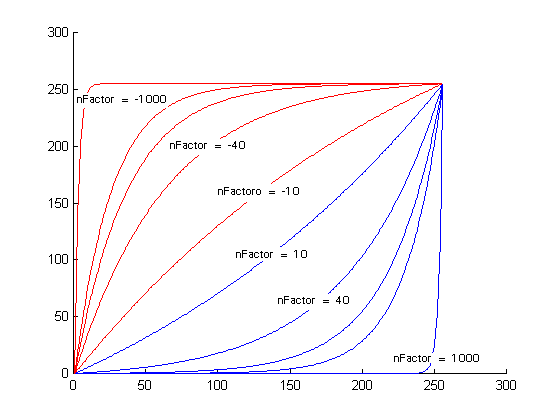If uFlags is FLT_LN the value of `nFactor` modifies the lookup table values (see figure below) according to the following equations: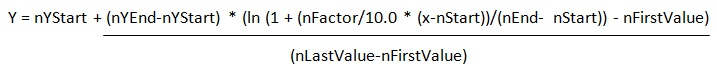Where:

• nYStart = The Lookup table value at nStart
• nYEnd = The Lookup table value at nEnd
• nFirstValue = 0
• nLastValue = ln(1 + nFactor/10.0)
• x = the intensity value of the selected pointIf `uFlags` is FLT_SIGMOID the value of nFactor modifies the lookup table values (see figure below) according to the following equations: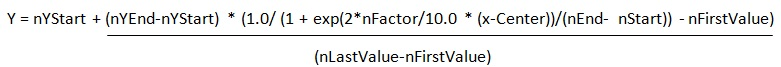Where:

• nYStart = The Lookup table value at nStart
• nYEnd = The Lookup table value at nEnd
• nFirstValue = 1.0/(1+exp(2.*nFactor/10.0*(nStart - Center)/ (nEnd- nStart)))
• nLastValue = 1.0/(1+exp(2.*nFactor/10.0*(nEnd - Center)/ (nEnd- nStart)))
• Center = (nEnd + nStart)/2
• x = the intensity value of the selected point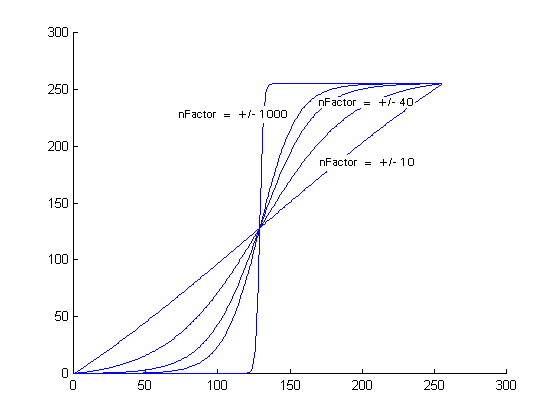If uFlags is FLT_LINEAR, `nFactor` is ignored. The function performs a linear interpolation between the two points (nStart, nYStart) and (nEnd, nYEnd) and stores the results in the lookup table. Where:

• nYStart = The Lookup table value at `nStart`
• nYEnd = The Lookup table value at `nEnd`

As an example, suppose a user wants to select a point, change the intensity value of that point (x), and then perform two linear interpolations:

• from 0 to the intensity value x
• from intensity value x to the end of the range

To accomplish this, the user would proceed by doing the following:

• Select the point and get its intensity value (x)
• Change the intensity value of the selected point to some new value (newIntensity).
• Store the new intensity value in the Lookup table by assignment:

````    pLookupTable = 0;   `
`    pLookupTable[x] = newIntensity; ````

• Call L_GetFunctionalLookupTable with nStart set to 0, nEnd set to x, and uFlags set to FLT_LINEAR.

• Store the new intensity value in the Lookup table by assignment:

````    pLookupTable[x] = newIntensity;//This can be ignored, because the lookup table should have the same value from the previous steps.   `
`    pLookupTable[nEnd] = uLookupLen; ````

• Call L_GetFunctionalLookupTable with nStart set to x, `nEnd` set to `uLookupLen`, and `uFlags` set to FLT_LINEAR. (For the example, assume the image is 8-bits per pixel.)

For another example, please refer to the example for L_RemapBitmapIntensity.

Required DLLs and Libraries

Platforms

Win32, x64, Linux.

See Also

## Example

This example will darken loaded bitmap by using lookup table affected by exponential function.

````L_INT GetFunctionalLookupTableExample(L_VOID) `
`{ `
`   L_INT nRet; `
`   L_UINT LookupTable;  /* Array to hold lookup table*/ `
`   BITMAPHANDLE LeadBitmap;  /*Bitmap handle to hold the loaded image */ `
` `
`   /* Load the bitmap, force 24 bits per pixel */ `
`   nRet = L_LoadBitmap (MAKE_IMAGE_PATH(TEXT("ImageProcessingDemo\\Image3.cmp")), &LeadBitmap, sizeof(BITMAPHANDLE), 24, ORDER_BGR, NULL, NULL);  `
`   if(nRet !=SUCCESS) `
`      return nRet; `
`   /*Get Lookup table where the array calculated by the exponential function for all the items of the array  from 0 - 255*/ `
`   nRet = L_GetFunctionalLookupTable ((L_INT *)LookupTable, 256,0, 255, 5, FLT_EXP); `
`   if(nRet !=SUCCESS) `
`      return nRet; `
`   nRet = L_RemapBitmapIntensity (&LeadBitmap, (L_INT *)LookupTable, 256,CHANNEL_MASTER); `
`   if(nRet !=SUCCESS) `
`      return nRet; `
`   nRet = L_SaveBitmap(MAKE_IMAGE_PATH(TEXT("Result.BMP")), &LeadBitmap, FILE_BMP, 24, 0, NULL); `
`   if(nRet !=SUCCESS) `
`      return nRet; `
`   //free bitmap  `
`   if(LeadBitmap.Flags.Allocated)   `
`      L_FreeBitmap(&LeadBitmap);   `
`   return SUCCESS; `
`} ````

Help Version 21.0.2021.4.7
Products | Support | Contact Us | Intellectual Property Notices
© 1991-2021 LEAD Technologies, Inc. All Rights Reserved.